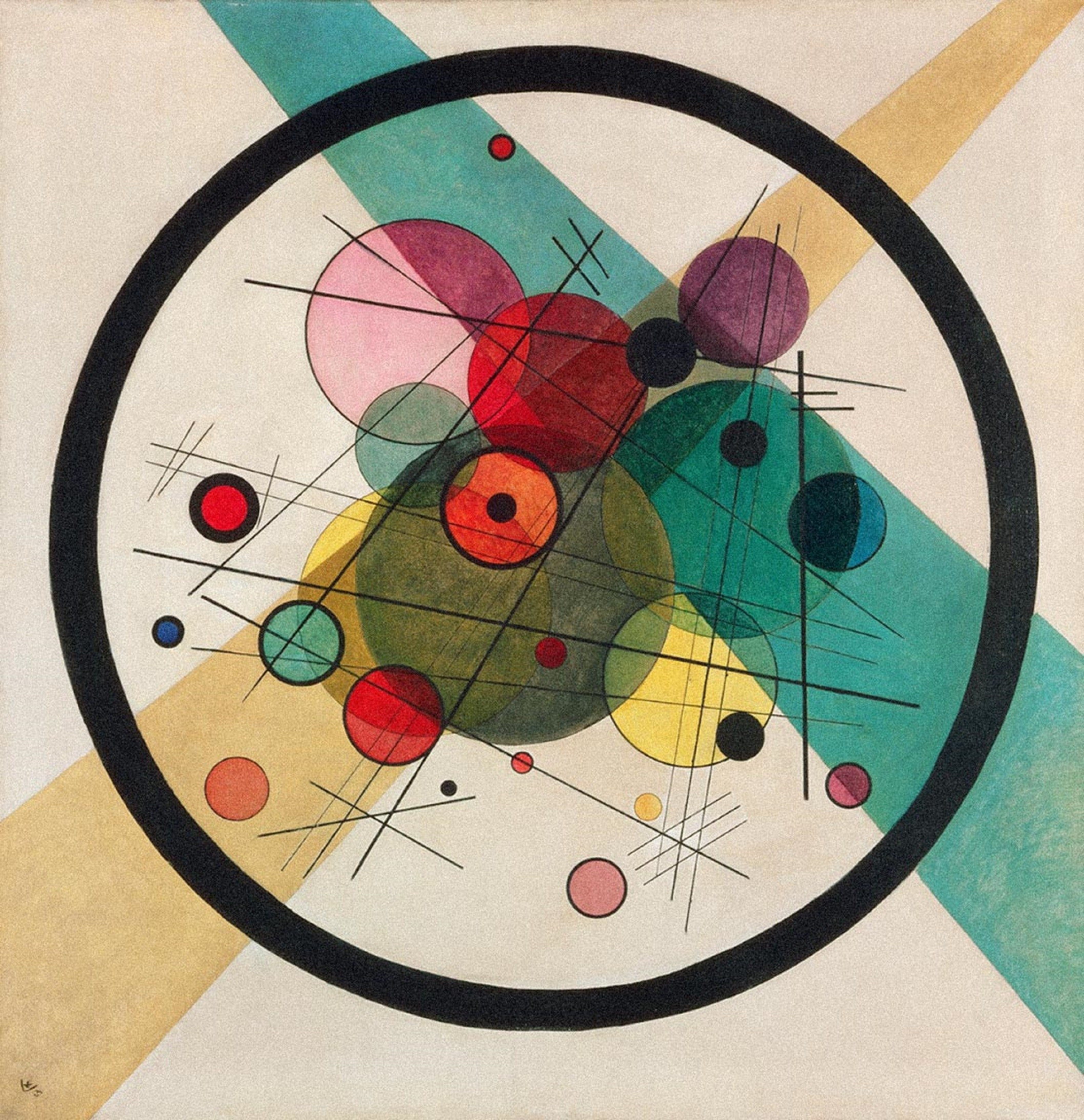# Dmitry VaintrobI am a Morrey Visiting Assistant Professor at UC Berkeley. I received my Ph. D. under Roman Bezrukavnikov at MIT. I currently work on problems at the intersection of logarithmic algebraic geometry, higher algebra, mirror symmetry and number theory. Since Summer of 2020 I have been running a learning seminar on QFT.

## Featured Papers:

1. Formality of little disks and algebraic geometry. Here I give a proof of formality of the little disks operad (as well as its cousin, the framed little disks operad) using logarithmic algebraic geometry, together with an explicit chain of quasiisomorphisms realizing this formality. This is the first purely algebro-geometric proof of this fact: all previous proofs had used transcendental techniques.
2. Moduli of framed formal curves. Here I study a moduli problem in formal algebraic geometry, and deduce a model in logarithmic algebraic geometry for certain "conformal" operads. In particular, this implies that the operads of little disks and the operad of framed little disks both are operads in an appropriate category of logarithmic motives (stably and with rational coefficients, this follows from a famous paper of Dmitry Tamarkin).
3. Categorical logarithmic Hodge theory. Here I define an unusually well-behaved category of quasicoherent sheaves on a logarithmic variety in terms of almost mathematics on the root stack. The new feature of this category is that in characteristic zero it has the "correct" Hochschild homology and cohomology. This implies in particular a noncommutative Hodge theory for log varieties in positive characteristic, something that has previously only been conjectured to exist. When applied to a toric variety, this gives a mirror for the category of all sheaves on the topological torus (with no constructibility condition).

A copy of my CV.